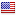#### Join the players club!

I see you dont have an account, join the club to get acces to everything! Make friends, keep track of scores, discover courses and more.## Bent creek

Par:
71
Total distance:
1568 Meters
Country:City:
Jacksonville
golf_course Hole 1 2 3 4 5 6 7 8 9 10 11 12 13 14 15 16 17 18 Total
Par 5 4 3 3 4 3 4 5 4 4 4 4 3 5 4 5 3 4 71
Distance 128m 96m 60m 52m 96m 71m 72m 96m 74m 89m 95m 90m 66m 125m 87m 112m 73m 86m 1568m
Strokeindex 0 0 0 0 0 0 0 0 0 0 0 0 0 0 0 0 0 0 -

### Last 10 rounds

• Date
Course
Description
• ```stdClass Object
(
[by] => stdClass Object
(
)

[coursename] =>  Bent creek
[course] => stdClass Object
(
[_id] => 590b266df509271ce1650c31
[name] =>  Bent creek
[footgolfclub] =>
[country] => US
[city] => Jacksonville
[total] => stdClass Object
(
[par] => 71
[meters] => 1567.51
)

[holes] => Array
(
 => stdClass Object
(
[par] => 5
[meters] => 127.96
[strokeindex] => 0
)

 => stdClass Object
(
[par] => 4
[meters] => 95.97
[strokeindex] => 0
)

 => stdClass Object
(
[par] => 3
[meters] => 60.324
[strokeindex] => 0
)

 => stdClass Object
(
[par] => 3
[meters] => 52.098
[strokeindex] => 0
)

 => stdClass Object
(
[par] => 4
[meters] => 95.97
[strokeindex] => 0
)

 => stdClass Object
(
[par] => 3
[meters] => 71.292
[strokeindex] => 0
)

 => stdClass Object
(
[par] => 4
[meters] => 72.206
[strokeindex] => 0
)

 => stdClass Object
(
[par] => 5
[meters] => 95.97
[strokeindex] => 0
)

 => stdClass Object
(
[par] => 4
[meters] => 74.034
[strokeindex] => 0
)

 => stdClass Object
(
[par] => 4
[meters] => 88.658
[strokeindex] => 0
)

 => stdClass Object
(
[par] => 4
[meters] => 95.056
[strokeindex] => 0
)

 => stdClass Object
(
[par] => 4
[meters] => 89.572
[strokeindex] => 0
)

 => stdClass Object
(
[par] => 3
[meters] => 65.808
[strokeindex] => 0
)

 => stdClass Object
(
[par] => 5
[meters] => 125.218
[strokeindex] => 0
)

 => stdClass Object
(
[par] => 4
[meters] => 86.83
[strokeindex] => 0
)

 => stdClass Object
(
[par] => 5
[meters] => 111.508
[strokeindex] => 0
)

 => stdClass Object
(
[par] => 3
[meters] => 73.12
[strokeindex] => 0
)

 => stdClass Object
(
[par] => 4
[meters] => 85.916
[strokeindex] => 0
)

)

[numholes] => 18
[first9] => stdClass Object
(
[par] => 35
[meters] => 745.824
)

[second9] => stdClass Object
(
[par] => 36
[meters] => 821.686
)

[logo] => true
[photo] => true
[player] => stdClass Object
(
[ObjectId] => 58d3a1c9f509270d1c5c8d02
)

[metrics] => yards
[active] => true
[email] => true
)

[description] => Best Round
[roundtype] => training
[flight] => Array
(
 => stdClass Object
(
[personName] => Nick Richardson
[photo] =>
[holes] => Array
(
 => stdClass Object
(
[round] =>
[course] => 590b266df509271ce1650c31
[hole] => 0
[holepar] => 5
[scoretrack] => -2
[score] => 3
)

 => stdClass Object
(
[round] =>
[course] => 590b266df509271ce1650c31
[hole] => 1
[holepar] => 4
[scoretrack] => -2
[score] => 2
)

 => stdClass Object
(
[round] =>
[course] => 590b266df509271ce1650c31
[hole] => 3
[holepar] => 3
[scoretrack] => -2
[score] => 1
)

 => stdClass Object
(
[round] =>
[course] => 590b266df509271ce1650c31
[hole] => 4
[holepar] => 4
[scoretrack] => -1
[score] => 3
)

 => stdClass Object
(
[round] =>
[course] => 590b266df509271ce1650c31
[hole] => 5
[holepar] => 3
[scoretrack] => 0
[score] => 3
)

 => stdClass Object
(
[round] =>
[course] => 590b266df509271ce1650c31
[hole] => 6
[holepar] => 4
[scoretrack] => -1
[score] => 3
)

 => stdClass Object
(
[round] =>
[course] => 590b266df509271ce1650c31
[hole] => 7
[holepar] => 5
[scoretrack] => -2
[score] => 3
)

 => stdClass Object
(
[round] =>
[course] => 590b266df509271ce1650c31
[hole] => 8
[holepar] => 4
[scoretrack] => -2
[score] => 2
)

 => stdClass Object
(
[round] =>
[course] => 590b266df509271ce1650c31
[hole] => 9
[holepar] => 4
[scoretrack] => -1
[score] => 3
)

 => stdClass Object
(
[round] =>
[course] => 590b266df509271ce1650c31
[hole] => 10
[holepar] => 4
[scoretrack] => -1
[score] => 3
)

 => stdClass Object
(
[round] =>
[course] => 590b266df509271ce1650c31
[hole] => 11
[holepar] => 4
[scoretrack] => -1
[score] => 3
)

 => stdClass Object
(
[round] =>
[course] => 590b266df509271ce1650c31
[hole] => 12
[holepar] => 3
[scoretrack] => 0
[score] => 3
)

 => stdClass Object
(
[round] =>
[course] => 590b266df509271ce1650c31
[hole] => 13
[holepar] => 5
[scoretrack] => -2
[score] => 3
)

 => stdClass Object
(
[round] =>
[course] => 590b266df509271ce1650c31
[hole] => 14
[holepar] => 4
[scoretrack] => -1
[score] => 3
)

 => stdClass Object
(
[round] =>
[course] => 590b266df509271ce1650c31
[hole] => 15
[holepar] => 5
[scoretrack] => -1
[score] => 4
)

 => stdClass Object
(
[round] =>
[course] => 590b266df509271ce1650c31
[hole] => 16
[holepar] => 3
[scoretrack] => 0
[score] => 3
)

 => stdClass Object
(
[round] =>
[course] => 590b266df509271ce1650c31
[hole] => 17
[holepar] => 4
[scoretrack] => -1
[score] => 3
)

 => stdClass Object
(
[round] =>
[course] => 590b266df509271ce1650c31
[hole] => 2
[holepar] => 3
[scoretrack] => 0
[score] => 3
)

)

[country] => US
[totals] => stdClass Object
(
[totalScore] => -20
[totalShots] => 51
)

)

)

[open] =>
[startdate] => stdClass Object
(
[\$date] => 1461172680000
[\$unixtime] => 1461172680
[_datetime] => 2016-04-20 19:18:00
)

[numholes18] =>
)
```